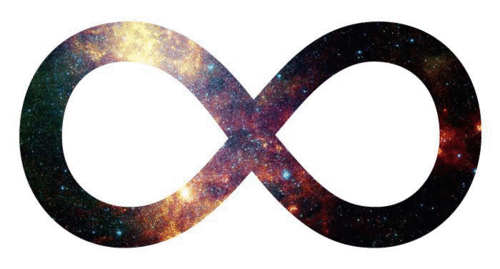# Is Infinity Real?

There is an interesting discussion at Quanta “Solution: ‘Is Infinity Real?’” – Is infinity a real physical phenomenon outside our models? Max Tegmark doesn’t think so – while admitting it is indisputably useful for mathematical models of physics, he believes that nothing is truly continuous – including space and time.Would an infinitely X* phenomenon be amenable to observational evidence? Perhaps not – and if so, we can never count one infinity, making it difficult to assign a likelihood that infinity exists in the territory and not as just convenient approximations in our maps.
Max believes also there are good philosophical reasons to ditch infinity and pitfalls in assuming infinity in mathematical models. Four points that should be understood (which are detailed in the linked Quanta article):
1. The map is not the territory.
2. Infinity is valid in mathematical models and can be very useful.
3. In the physical world, there are compelling practical and philosophical reasons to reject infinity as a default assumption.
4. There will be limiting cases where the mathematical infinity assumption and the physical absence of infinity result in different answers.

Finite models are proposed as solutions to replace infinite solutions for a few mathematical problems: Hilbert’s hotel, the 100, 200, 300 Triangle, and the Elliptical Pool Table.
“So the bottom line is: Infinity is permissible in mathematics applied to physics because it makes things convenient and tractable in most cases. However, we must be alert for limiting cases where our models are bound to fail, and we will then need to apply different methods.”

*X could represent huge, small, powerful etc..

I had a discussion about this with a friend Adam Karlovsky – and I was surprised when this just came up on my radar – it’s an interesting read.  We discussed the possibility that infinite randomness would produce an infinite amount of copies of Adam Karlovsky – doing an infinite amount of things.  He said that at one stage this thought kept him up at night.  I have had my doubts about the realism of infinity.

So what do you think?

Is Infinity Real?

View ResultsLoading ...